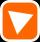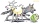# MO Z8–I–6 2018

In the KLMN trapeze, KL has a 40 cm base and an MN of 16 cm. Point P lies on the KL line so that the NP segment divides the trapezoid into two parts with the same area. Find the length of the KP line.

Result

x =  28 cm

#### Solution:Leave us a comment of example and its solution (i.e. if it is still somewhat unclear...):

Showing 0 comments:Be the first to comment!#### To solve this verbal math problem are needed these knowledge from mathematics:

Looking for help with calculating arithmetic mean? Looking for a statistical calculator? See also our trigonometric triangle calculator.

## Next similar examples:

1. Trapezium basesFind the trapezium height if a = 8 cm and c = 4 cm if its content 21 square centimeters.
2. Parcelparcel has a rectangular shape of a trapezoid with bases 12 m and 10 m and a height 8 m. On parcel was built object with a footprint an isosceles triangle shape with side 4 m and height three-quarters of a meter. What is the area of unbuild parcel?
3. TrapeziumThe area of trapezium is 35cm2 find its altitude if the bases are 6cm and 8cm.
4. Roof tilesThe roof has a trapezoidal shape with bases of 15 m and 10 m, height of roof is 4 meters. How many tiles will need if on 1 m2 should be used 8 tiles?
5. Trapezoid ABCDABDC is a trapezoid in which AB and CD are parallel sides measuring 6 and 9 respectively. Angles ABC and BCD are both right angles. Find the length of segment BD.
6. Area of ditchHow great content area will have a section of trapezoidal ditch with a width of 1.6 meters above and below 0.57 meters? The depth of the ditch is 2.08 meters.
7. Trapezium 2Trapezium has an area of 24 square cms. How many different trapeziums can be formed ?
8. Cross sectionThe cross-section ABCD of a swimming pool is a trapezium. Its width AB=14 meters, depth at the shallow end is 1.5 meters and at the deep end is 8 meter. Find the area of the cross-section.
9. A trapezoidA trapezoid 75 ft wide on top 85 ft on the bottom, the height is 120 ft. What is its area in the square yds?
10. Areaf of STIt is given square DBLK with side |BL|=13. Calculate area of triangle DKU if vertex U lie on line LB.
11. Center traverseIt is true that the middle traverse bisects the triangle?
12. RhombusPQRS is a rhombus. Given that PQ=3 cm & height of rhombus is given 2 cm. Calculate its area.
13. SimilarityABC is a triangle wherein a = 4 cm, b = 6 cm, c = 8 cm. Is it similar to the triangle DEF: d = 3 cm, e = 4.5 cm, f = 6 cm? If so, determine the ratio of similarity.
14. AverageThe arithmetic mean of the two numbers is 71.7. One number is 5. Calculate the second number.
15. MO 2016 Numerical axisCat's school use a special numerical axis. The distance between the numbers 1 and 2 is 1 cm, the distance between the numbers 2 and 3 is 3 cm, between the numbers 3 and 4 is 5 cm and so on, the distance between the next pair of natural numbers is always in
16. An equilateralAn equilateral triangle with a side 10 m represents a wooden platform standing in a lawn. A goat is tied to a corner with a 15 m rope. What is the maximum amount of grazing area available to the goat?
17. Pipe cross sectionThe pipe has an outside diameter 1100 mm and the pipe wall is 100 mm thick. Calculate the cross section of this pipe.